﻿ 基于ALE方法的平底结构入水冲击动力特性研究
 舰船科学技术2017, Vol. 39Issue (12): 125-133PDF

1. 华中科技大学 船舶与海洋工程学院，湖北 武汉 430074;
2. 武汉第二船舶设计研究所，湖北 武汉 430064;
3. 中国舰船研究设计中心，湖北 武汉 430064

Research on impact characteristics of water entry of a flat-bottom structure based on ALE method
YANG Li1, ZHOU Yong2, LI Hua-feng2, WANG Kang3, ZHANG Yong-ou1, LIU Tu-guang1
1. School of Naval Architecture and Ocean Engineering, Huazhong University of Science and Technology, Wuhan 430074, China;
2. Wuhan Second Ship Design and Research Institute, Wuhan 430064, China;
3. China Ship Development and Design Center, Wuhan 430064, China
Abstract: As a typical fluid-structure coupling problem, structure impacting into water is widely used in the field of ship and ocean engineering, especially as a flat model of offshore platform. It has important engineering application background and academic research value. In this paper, the ALE algorithm is used to simulate and analyze the two-dimensional elastic flat-bottomed structures under constant velocity impact. The Lagrange element and Euler element are used to characterize the structure and fluid. The simulation of the fluid-solid coupling process is realized by the coupling algorithm. The influence of air cushion on the bottom pressure of the structure, the conditions of mass, stiffness and water velocity are discussed. The results show that the pressure at the bottom edge of the flat bottom structure first reaches the peak value, and then the pressure peak appears in the direction of the width direction, and the motion after the constant velocity impact into the water is free vibration. The peak value of impact pressure increases with the increase of structure mass and stiffness, and the peak value of shock pressure has a linear relationship with velocity, increases with the increase of velocity, and the effect of the bottom angle on the peak value of impact pressure is significant. The above study provides an important basis for structural strength design in engineering application.
Key words: flat-bottom     structure impact into water     fluid-structure interaction     ALE algorithm
0 引　言

21世纪以来，随着计算机技术的发展，人们开始通过数值仿真方法研究结构入水问题。卢炽华等研究了二维弹性结构的入水冲击问题的数值方法，通过耦合有限元方程和边界元方程来求解流场和结构动力响应的相互作用的运动方程。夏斌等对平底结构入水问题进行仿真研究，讨论了冲击速度、底部平板厚度、弹性模量与弯曲刚度对结构冲击压力峰值的影响，并通过正交实验分析了各参数对冲击压力峰值的影响程度。进一步，陈震等利用MSC. Dytran模拟出空气层与水面的混合情况，并对空气垫的形成、底部压力峰值及分布作了详尽分析，还基于神经网络方法对底部冲击压力作出预报。Matej Vesenjak等基于LS-DYNA评估了不同的方法对简易容器盒里流体晃荡问题的计算结果，并与试验观测结果进行对比。Oger等用无网格SPH（Smoothed Particle Hydrodynamics）方法模拟了二维楔形结构冲击入水的流固耦合过程。Gong等则对二维楔形结构冲击入水SPH模型的边界条件作出改进，通过在边界加上实体海绵层来模拟无反射边界条件。吴家鸣等利用Fluent软件的VOF（Volume of Fluid）方法模拟大型平底结构在一定高度自由下落冲击限制水域引起的三维流体动力现象。Hu等采用同样的方法在2013年海底作业工具入水过程数值仿真的研究报告中分析了二维平底模型匀速垂直进入静水的水动力问题。

1 基本理论 1.1 弹性平底结构的入水过程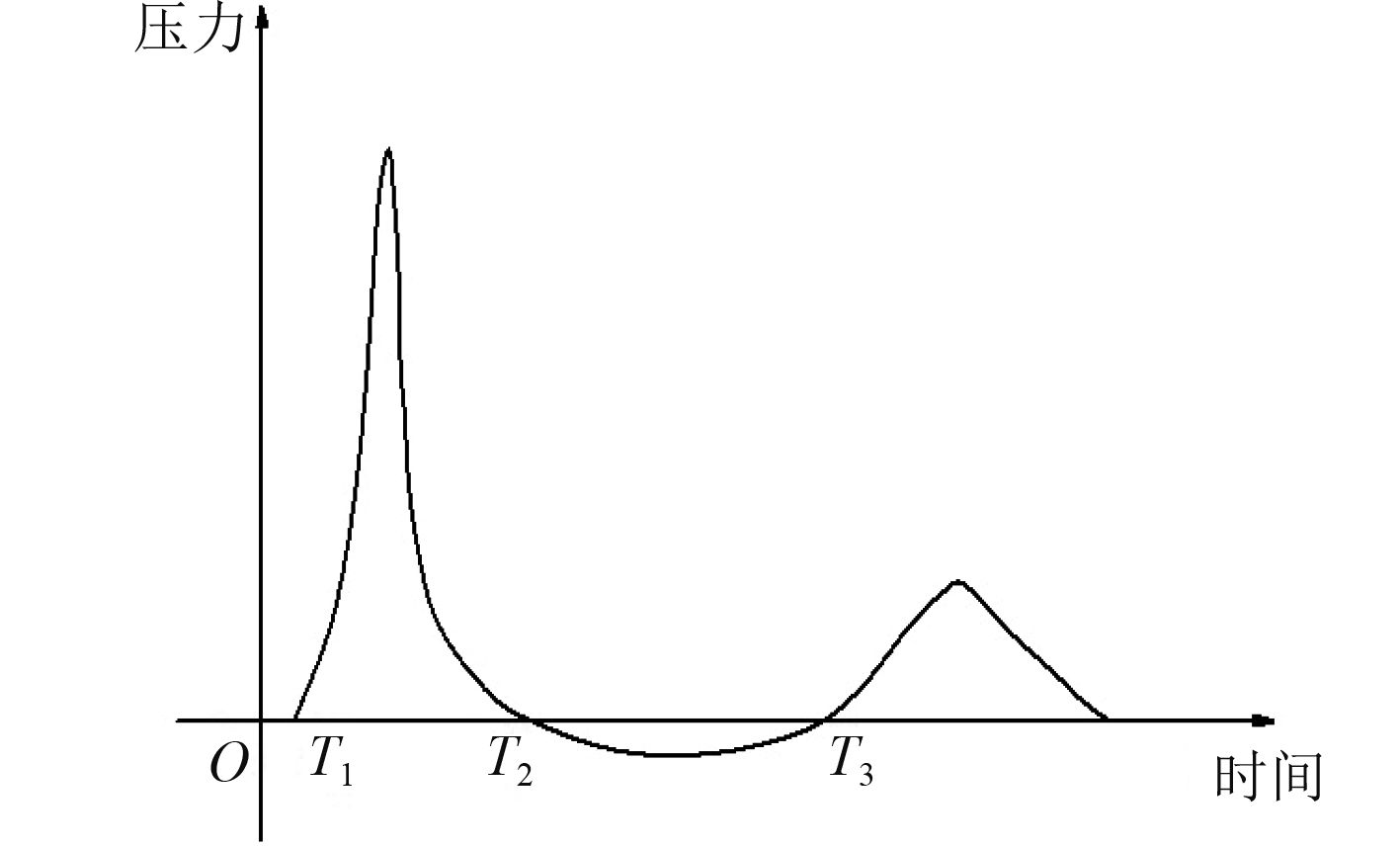图 1 弹性平底结构入水历程 Fig. 1 Water inlet course of elastic flat-bottom structure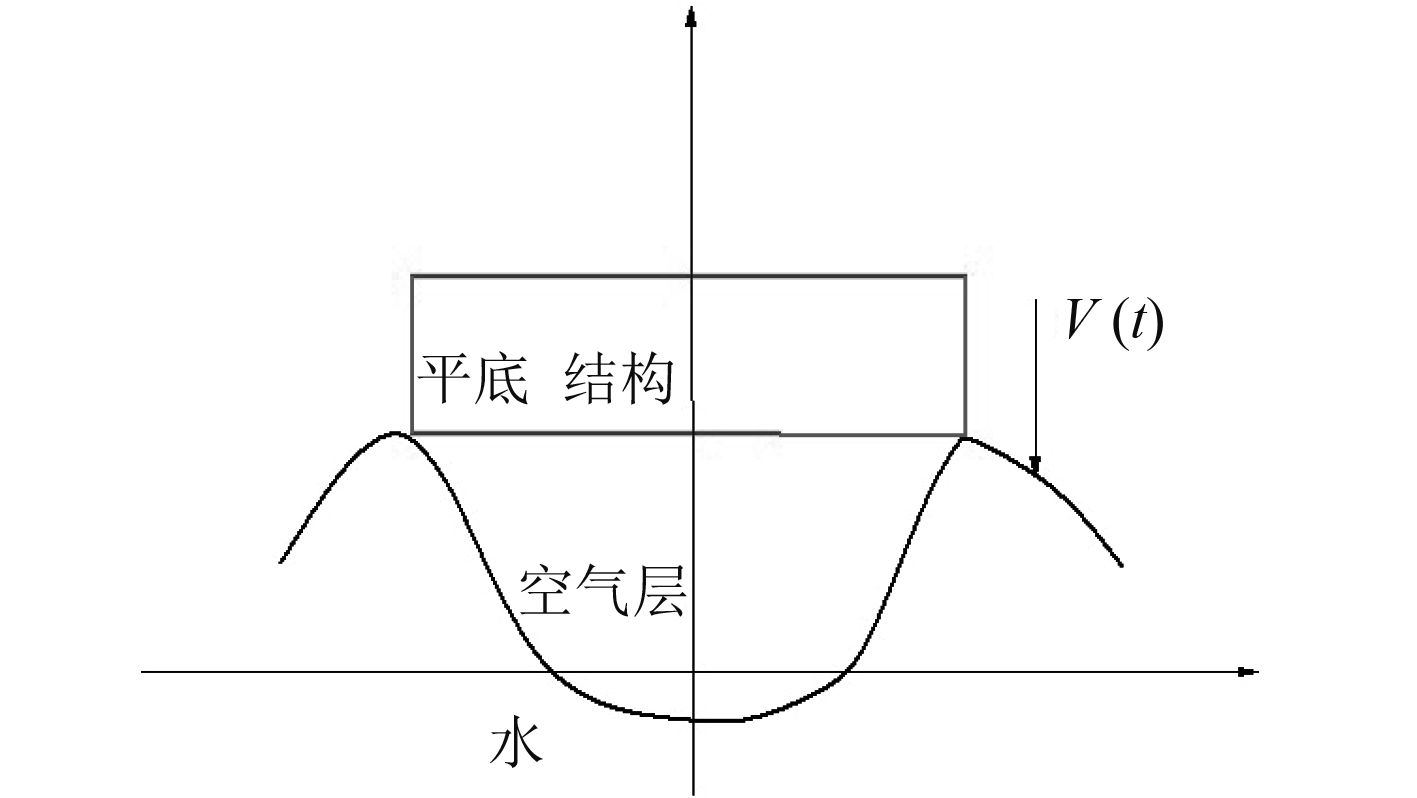图 2 平底结构的空气垫理论模型 Fig. 2 Theoretical model of air cushion with flat-bottom structure
1.2 ALE方法 1.2.1 ALE方法的理论基础

 $\frac{{\partial u}}{{\partial t}} + u\nabla u - 2{u^F}\nabla \varepsilon (u) + \nabla p = b{\text{，}}$ (1)
 $\nabla \cdot u = 0{\text{，}}$ (2)

 $\sigma = - pl + 2{v^F}\varepsilon (u){\text{，}}$ (3)
 $\varepsilon (u) = \frac{1}{2}(\nabla u + {(\nabla u)^{\rm{T}}}){\text{。}}$ (4)

 $\frac{{\partial f({X_i},t)}}{{\partial t}} = \frac{{\partial f({x_i},t)}}{{\partial t}} + {w_i}\frac{{\partial f({x_i},t)}}{{\partial {x_i}}}{\text{，}}$ (5)

1）质量守恒方程

 $\frac{{\partial \rho }}{{\partial t}} = - \rho \frac{{\partial {v_i}}}{{\partial {x_i}}} - {w_i}\frac{{\partial \rho }}{{\partial {x_i}}}{\text{。}}$ (6)

2）动量守恒方程

 $v\frac{{\partial {v_i}}}{{\partial t}} = {\sigma _{ij,j}} + \rho {b_i} - \rho {w_i}\frac{{\partial {v_i}}}{{\partial {x_j}}}{\text{。}}$ (7)

 ${\sigma _{ij}} = - p{\delta _{ij}} + \mu ({v_{i,j}} + {v_{j,i}}){\text{。}}$ (8)

 ${v_i} = U_i^0{\text{，}} {\text{在}}\varGamma 1{\text{域上}}{\text{，}}$ (9)
 ${\sigma _{ij}}{n_i} = 0{\text{，}} {\text{在}}\varGamma 2{\text{域上}}{\text{。}}$ (10)

 ${\varGamma _1}\bigcup {{\varGamma _2} = \varGamma } \;\;\;{\text{，}}{\varGamma _1}\bigcap {{\varGamma _2} = 0} {\text{。}}$ (11)

 ${v_i}({x_i},0) = 0{\text{。}}$ (12)

3）能量守恒方程

 $\rho \frac{{\partial E}}{{\partial t}} = {\sigma _{ij}}{v_{i,j}} + \rho {b_i}{v_i} - \rho {w_j}\frac{{\partial E}}{{\partial {x_j}}}{\text{。}}$ (13)

 $\rho \frac{{\partial {v_i}}}{{\partial t}} = {\sigma _{ij,j}} + \rho {b_i}{\text{，}}$ (14)
 $\rho \frac{{\partial E}}{{\partial t}} = {\sigma _{ij}}{v_{i,j}} + \rho {b_i}{v_i}{\text{。}}$ (15)

 ${u^{n + 1/2}} = {u^{n - 1/2}} + \Delta t{{M}^{ - 1}}({F_{\rm ext}}^n + {F_{{\mathop{\rm int}} }}^n){\text{，}}$ (16)
 ${x^{n + 1}} = {x^{n - 1}} + \Delta t{u^{n + 1/2}}{\text{。}}$ (17)

1.2.2 流固耦合算法

ALE算法。基本单元是定义一个流固耦合面即结构和流体的交界面，如图3所示，结构和流体分别采用Lagrange单元和Euler单元，通过约束条件将两者耦合起来，从而力学参量即可联系在一起。此算法中欧拉材料的流动产生的压载荷会自动作用到结构网格上，与此同时结构网格产生的变形又会影响欧拉材料的流动及对应的压力值。因此完全耦合的流体-结构响应可以用此方法表达。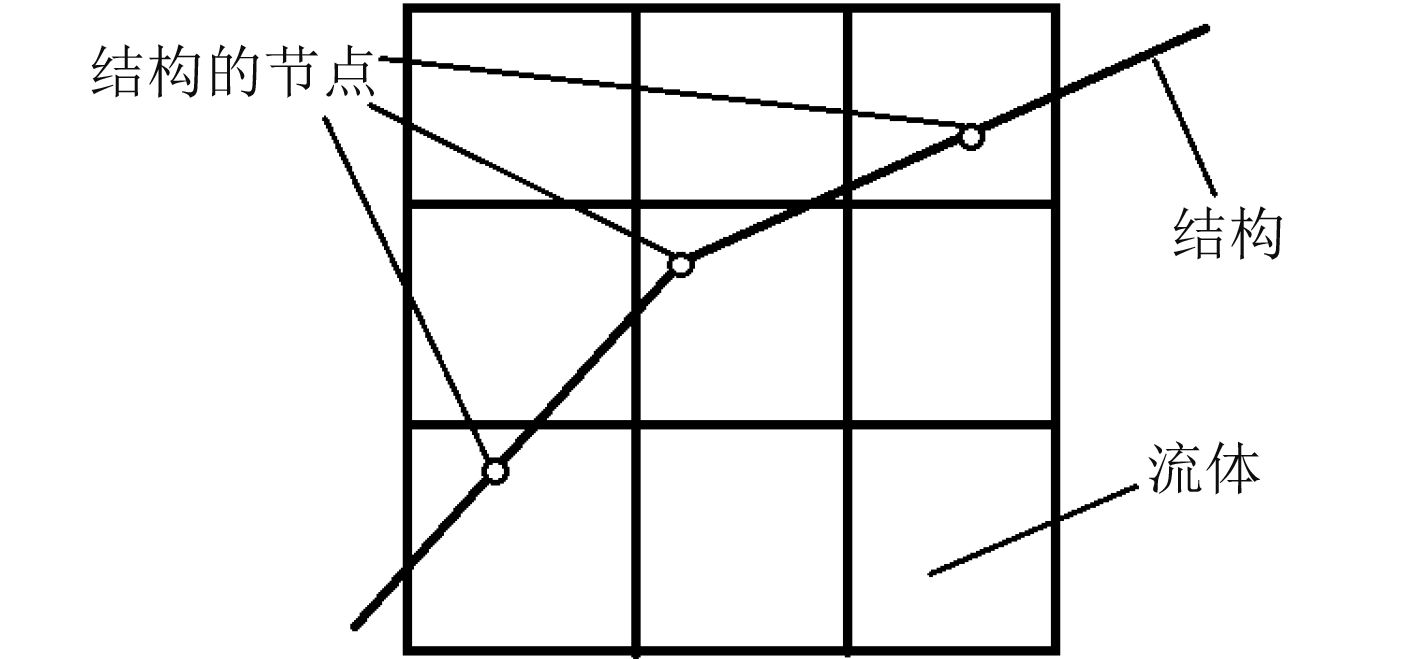图 3 ALE算法结构单元 Fig. 3 ALE algorithm structure unit
1.2.3 时间积分

 $\Delta t < \frac{{\Delta x}}{{c + u}}{\text{，}}$ (18)

 $\Delta t < \frac{{\Delta x}}{{Q + {{({Q^2} + {c^2})}^{1/2}}}}{\text{，}}$ (19)
 $\begin{array}{l}Q = {C_1}c + {C_2}\left| {div(u)} \right|;\\[7pt]div(u) < 0;\;Q = 0\;\;div(u) \geqslant 0{\text{。}}\end{array}$ (20)

 ${c_2} = \frac{{0.75G + k}}{{{\rho _o}}}{\text{，}}$ (21)
 $k = {\rho _o}\frac{{\partial P}}{{\partial \rho }} + \frac{P}{\rho }\frac{{\partial P}}{{\partial e}}{\text{。}}$ (22)

 $k = {\rho _o}{c^2}{\text{，}}$ (23)

 ${x^{n + 1}} = {x^n} + {u^{n + 1/2}}\Delta {t^n}{\text{，}}$ (24)
 ${u^{n + 1/2}} = {u^{n - 1/2}} + \frac{1}{2}{a^n}(\Delta {t^n} + \Delta {t^{n + 1}}){\text{。}}$ (25)

 ${u^{n + 1/2}} = {u^{n - 1/2}} + \frac{{{F_n}}}{{2{\mathit{\boldsymbol{M}}}}}(\Delta {t^n} + \Delta {t^{n + 1}}){\text{。}}$ (26)

 ${F^n} = {F_{{\mathop{\rm int}} }}^n + {F_{{\rm{ext}}}}^n{\text{，}}$ (27)

 ${F_{{\mathop{ int}} }}^n = \int\limits_V {x{B^t}} {\sigma ^n}d{\text{，}}$ (28)
 ${\sigma ^n} = - {P^n}{I_d} + {\sigma _d}^n{\text{。}}$ (29)

Lagrange结构（从物质）和Euler流体（主物质）间的相对位移d。检查每一个从节点相对于主物质表面的情况，如果不贯穿就无需做任何操作。如果贯穿，界面力F应分布至Euler流体物质的节点上。其中界面力F的大小与贯穿数量有如下关系：

 $F = {k_i} \cdot d{\text{。}}$ (30)

2 仿真模型 2.1 几何模型图 4 二维平底结构入水冲击模型 Fig. 4 Water impact model of two-dimensional flat-bottom structure
2.2 计算参数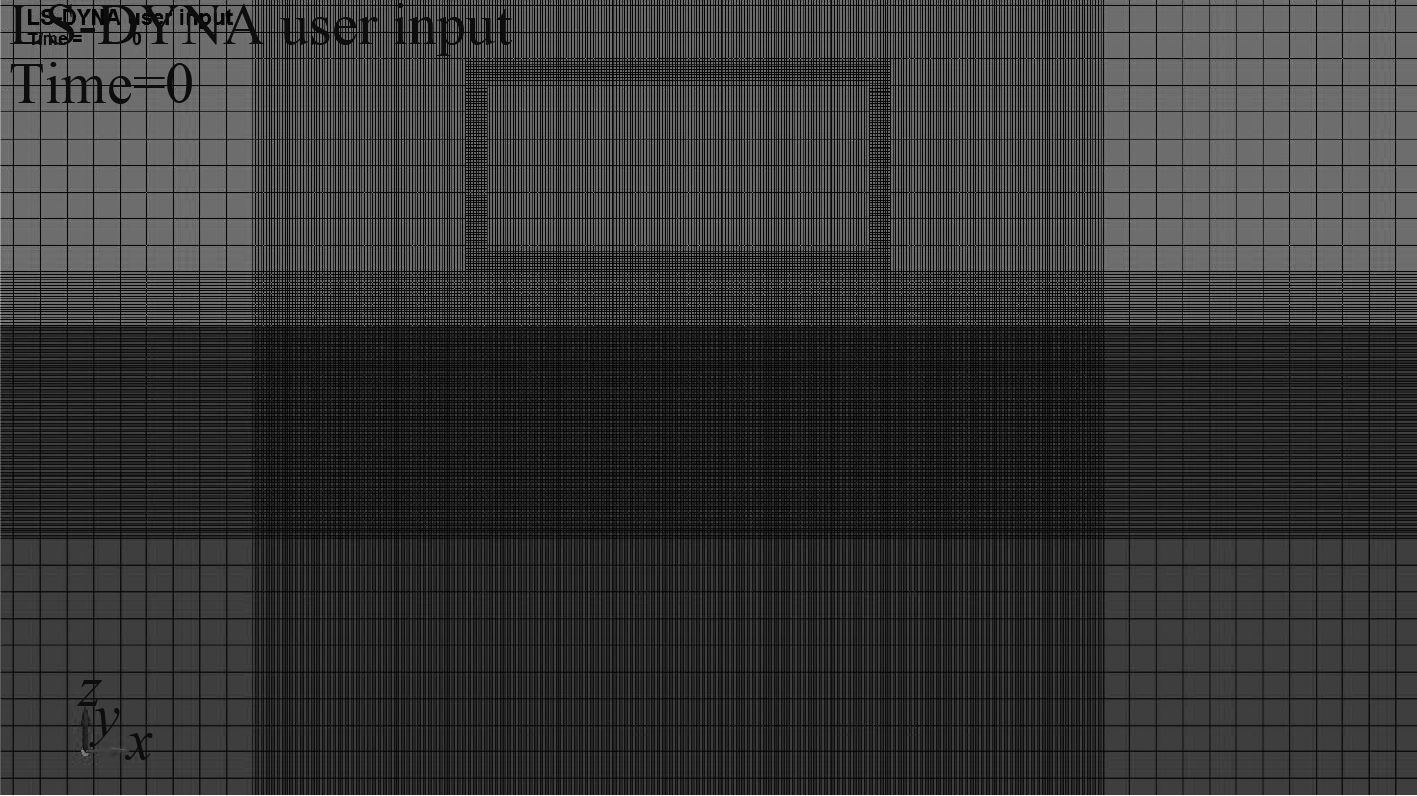图 5 网格划分 Fig. 5 Meshing

2.3 收敛性验证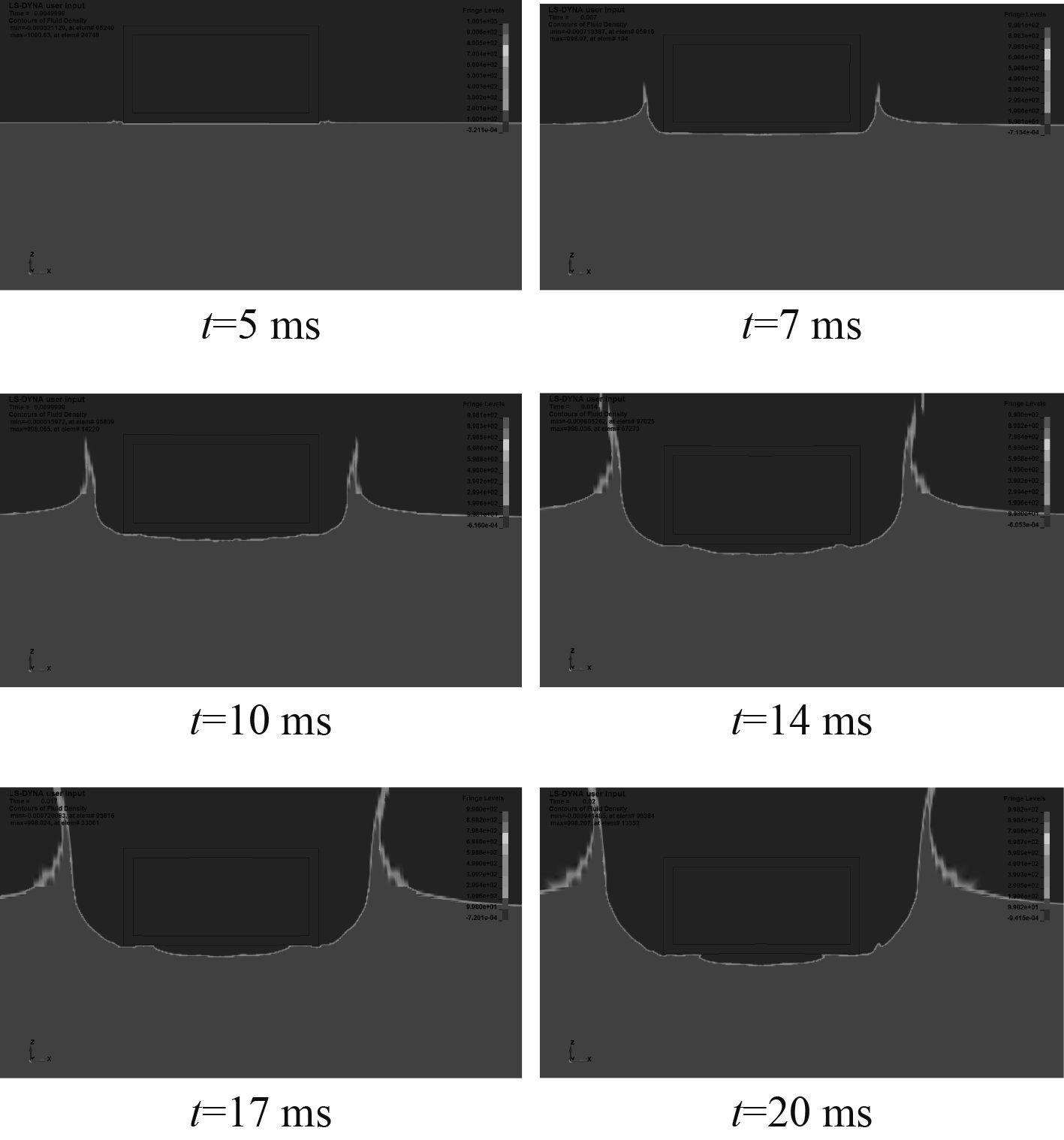图 6 ALE方法入水过程云图 Fig. 6 ALE method into the water process cloud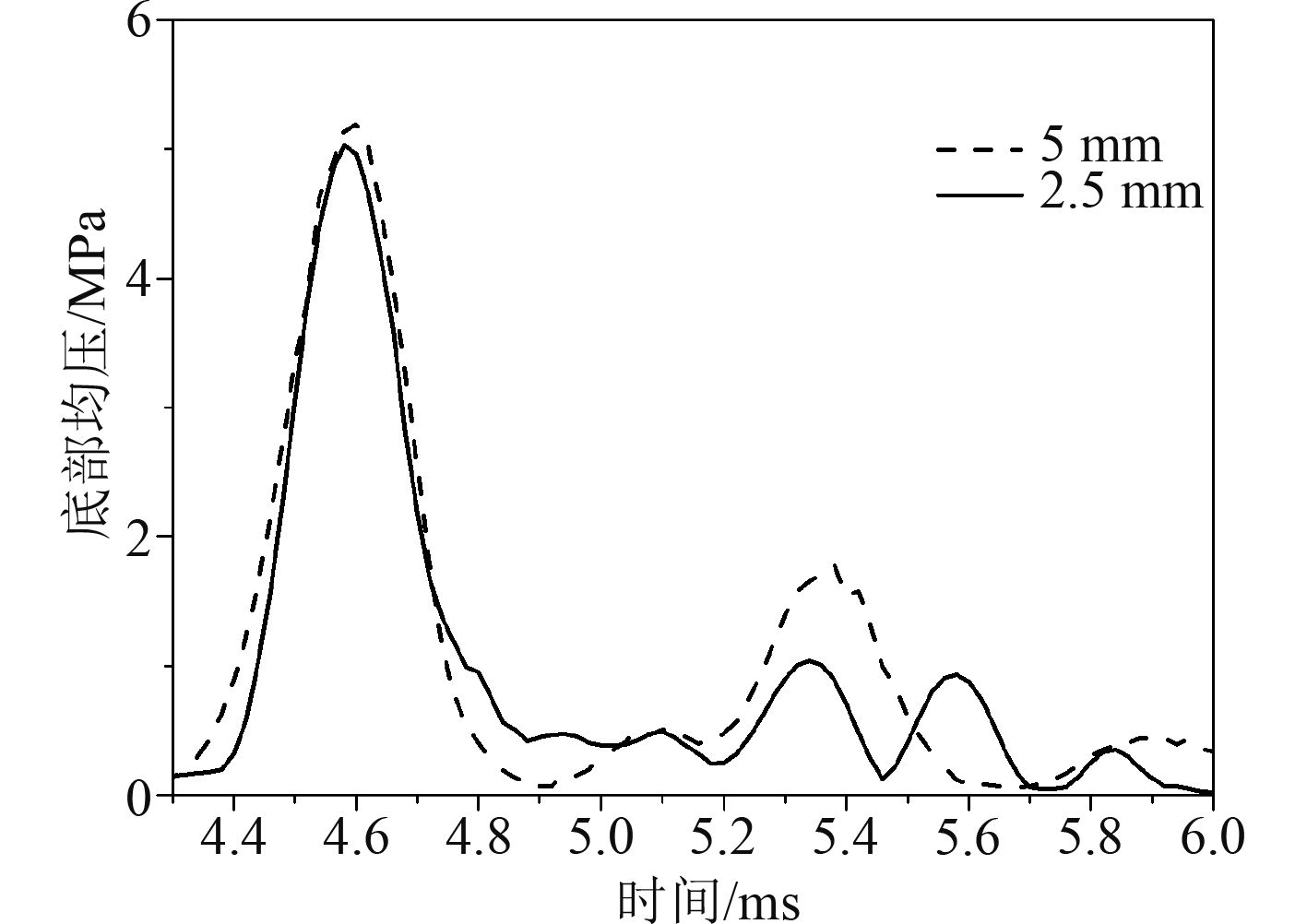图 7 5 mm网格和2.5 mm网格底部均压对比 Fig. 7 Comparison of 5 mm grid and 2.5 mm grid bottom pressure

3 结果分析 3.1 底部压力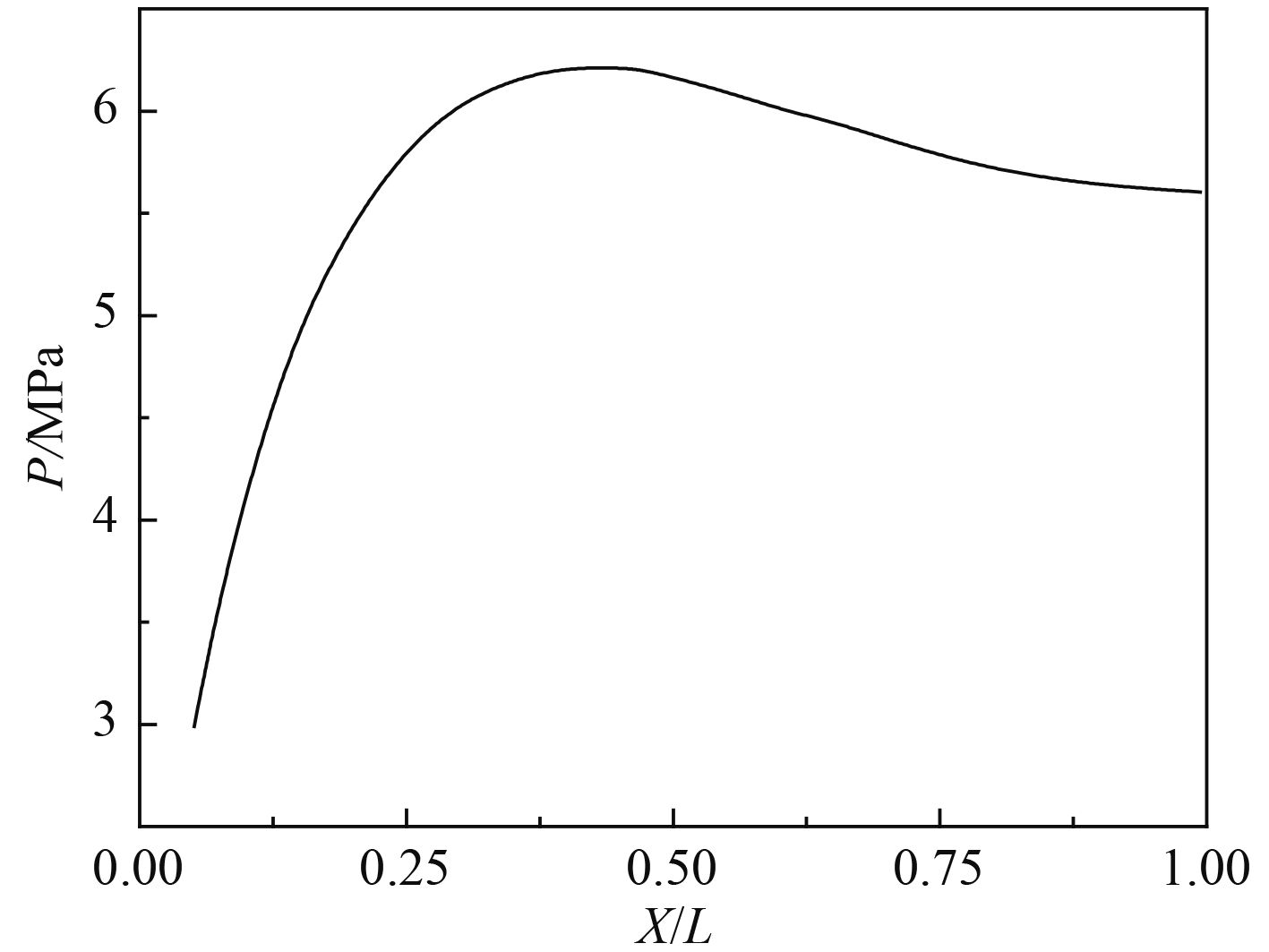图 8 底部压力分布 Fig. 8 Bottom pressure distribution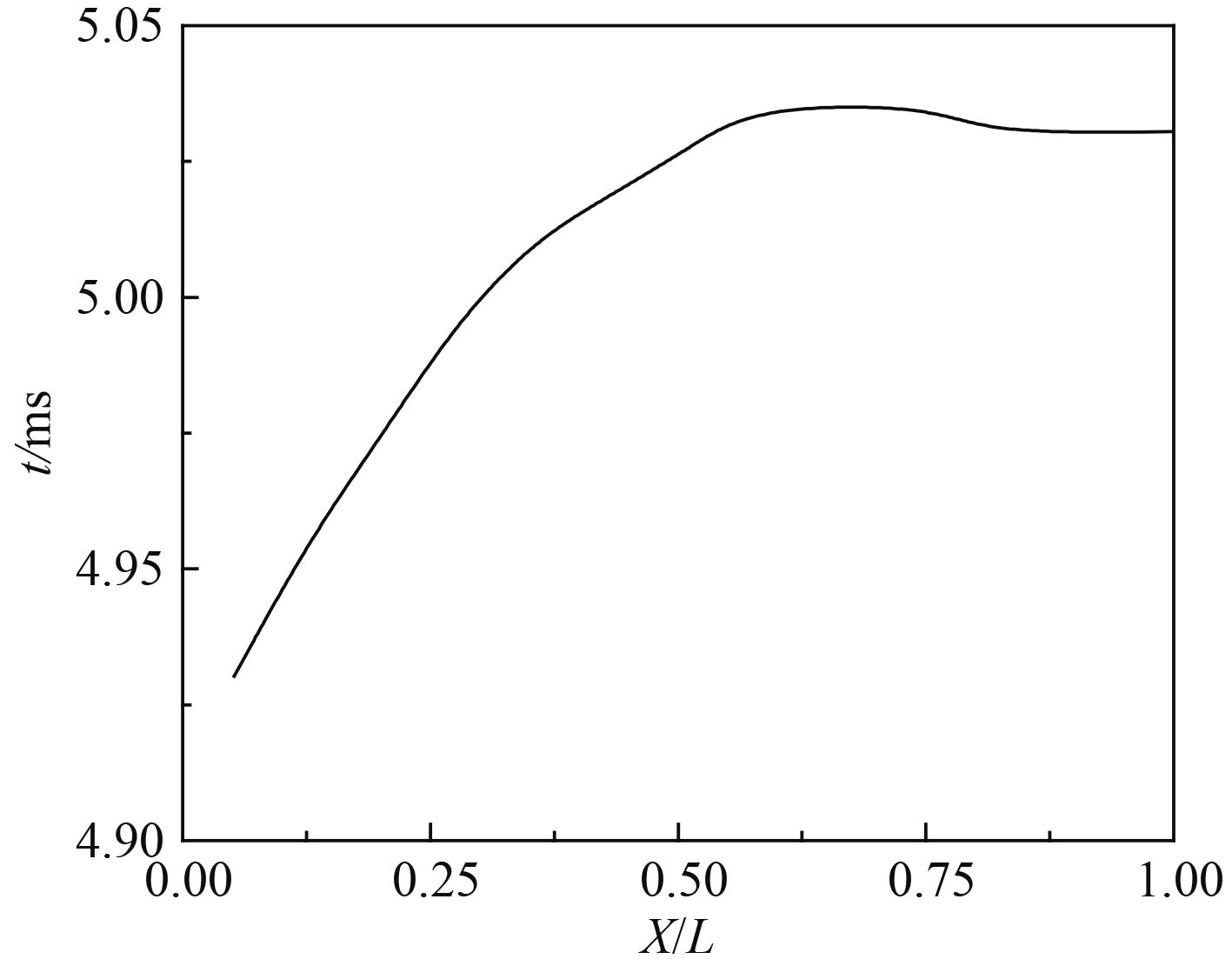图 9 所有观测点出现峰值的时刻 Fig. 9 The time of peak of all observation points

3.2 结构质量对冲击压力的影响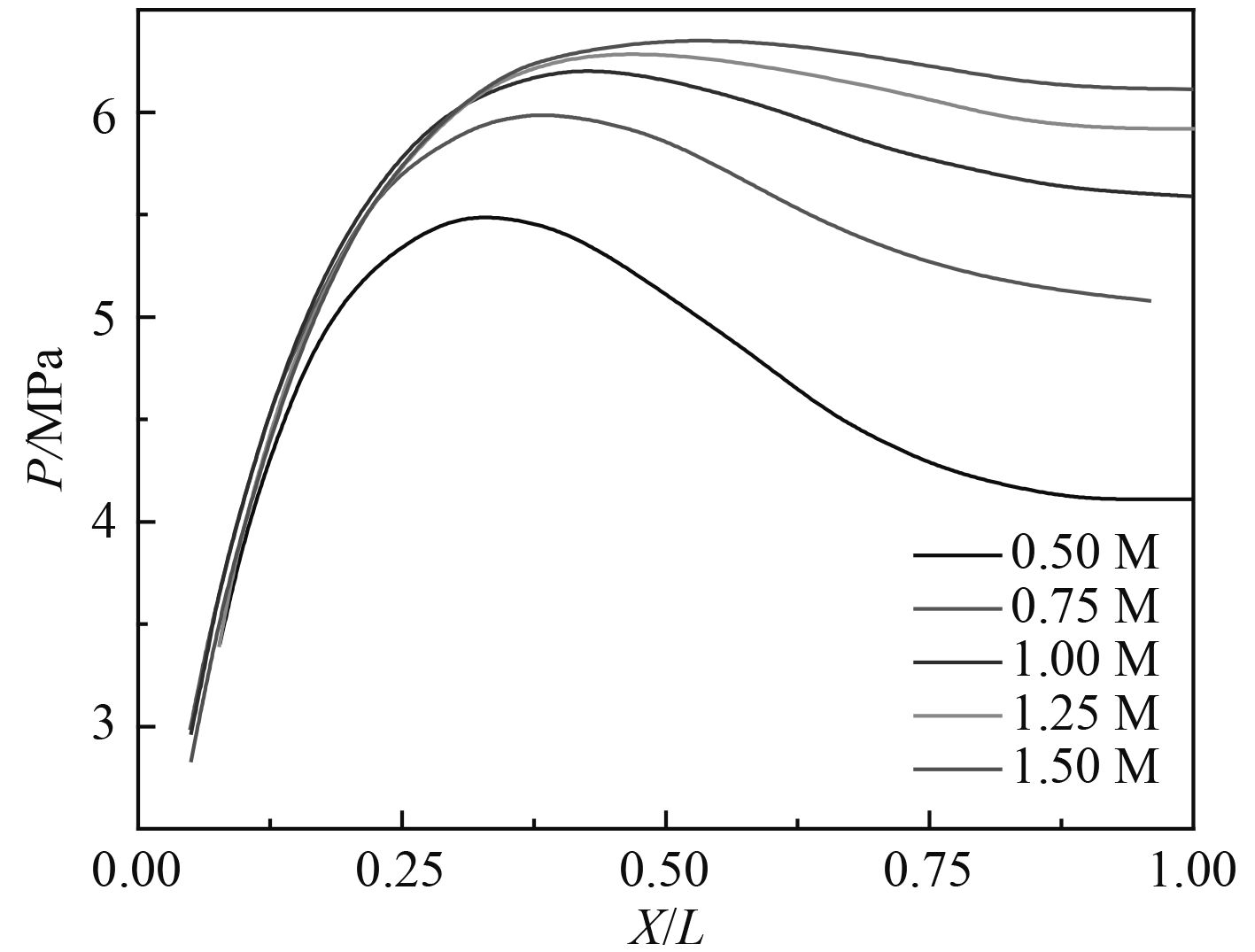图 10 质量对冲击压力的影响 Fig. 10 Effect of mass on pressure表 1 质量对冲击压力峰值的影响 Tab.1 Effect of mass on pressure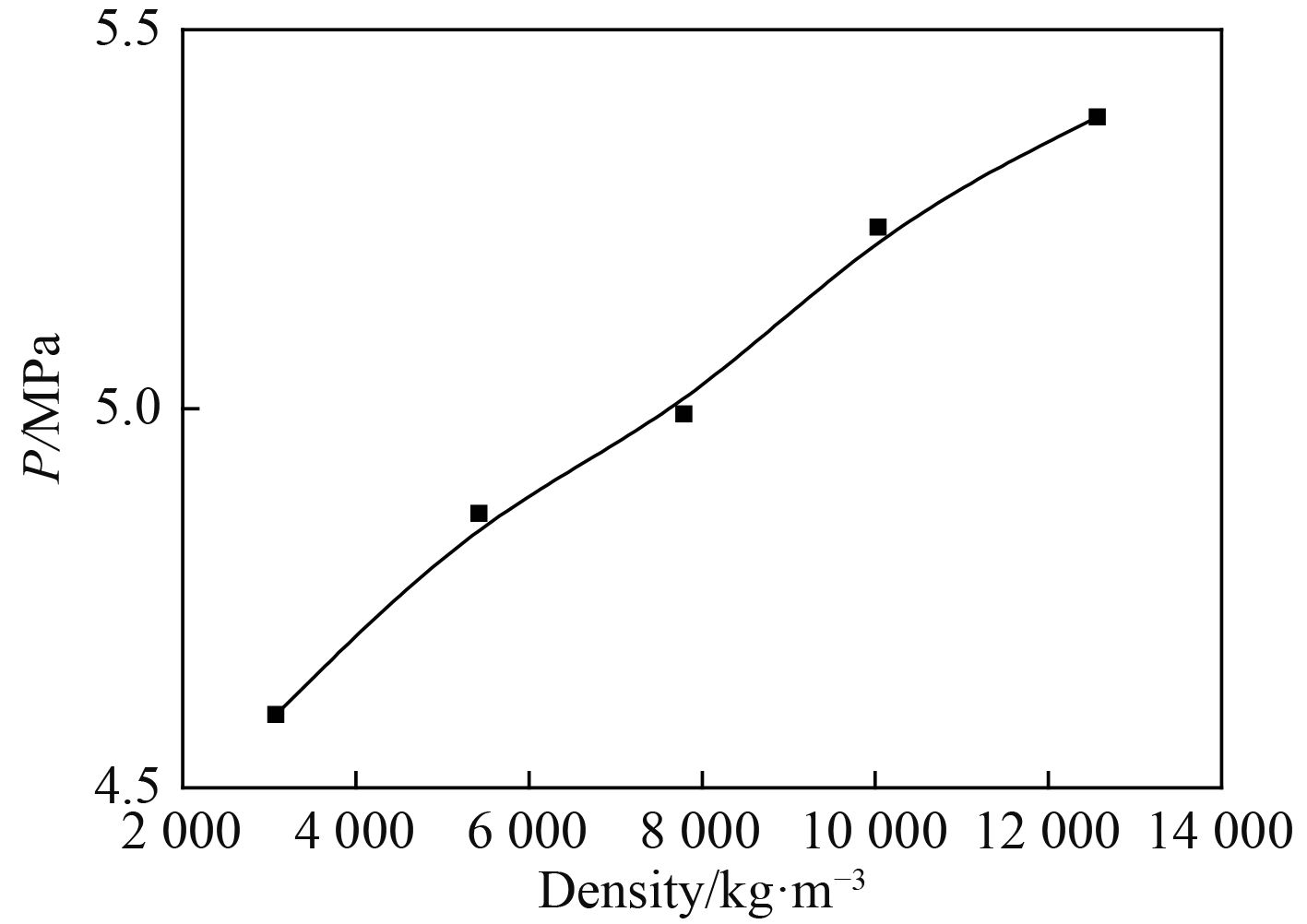图 11 结构密度对冲击压力的影响 Fig. 11 Effect of structural density on pressure
3.3 结构刚度对冲击压力的影响

 $D = \frac{{{{E}}{{{t}}^{\rm{3}}}}}{{{\rm{12}}\left( {{\rm{1 - }}{\mu ^{\rm{2}}}} \right)}}{\text{。}}$ (31)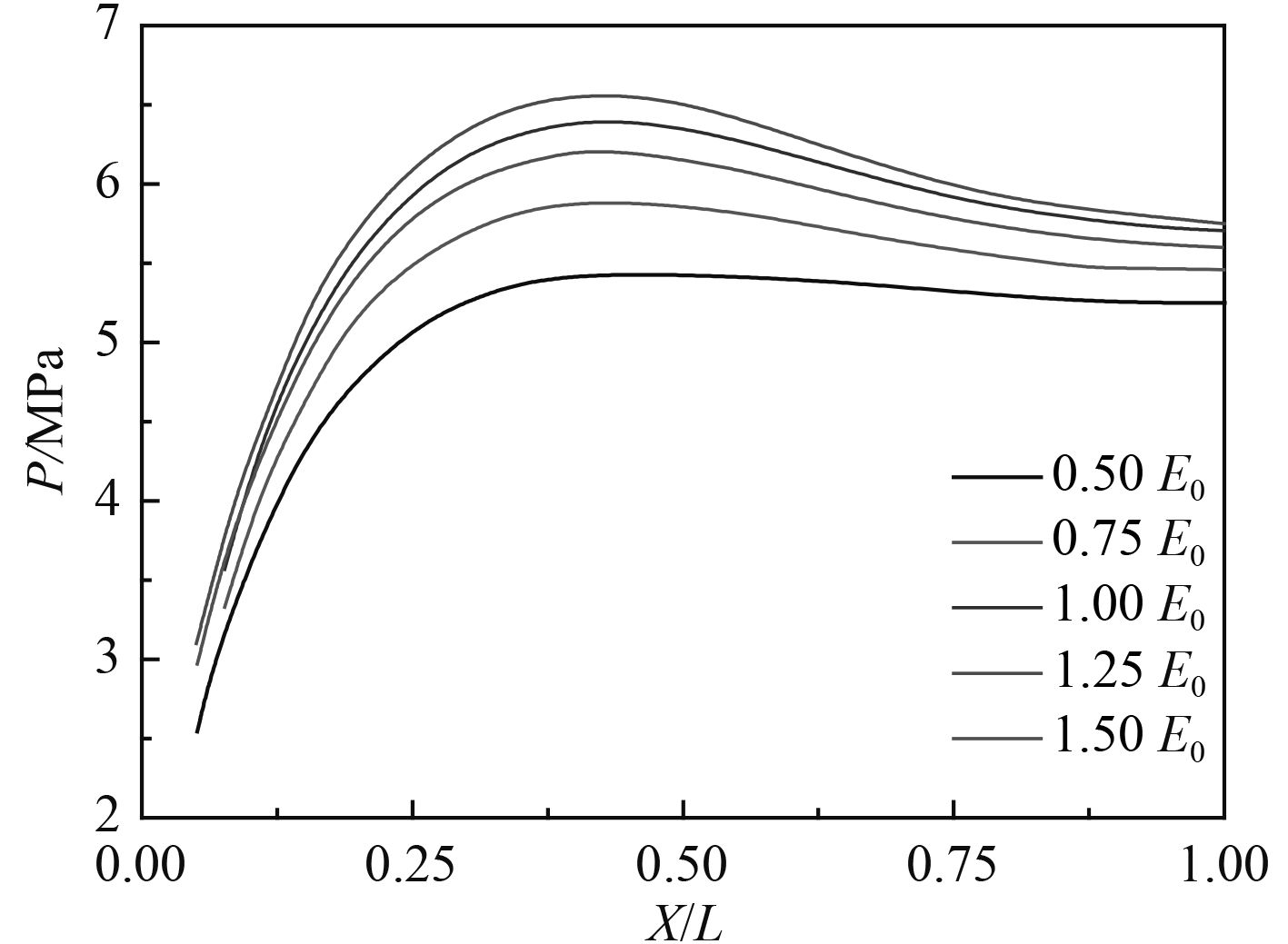图 12 不同弹性模量对冲击压力的影响 Fig. 12 Effect of different elastic moduli on pressure表 2 不同弹性模量对冲击压力的影响 Tab.2 Effect of different elastic moduls on pressure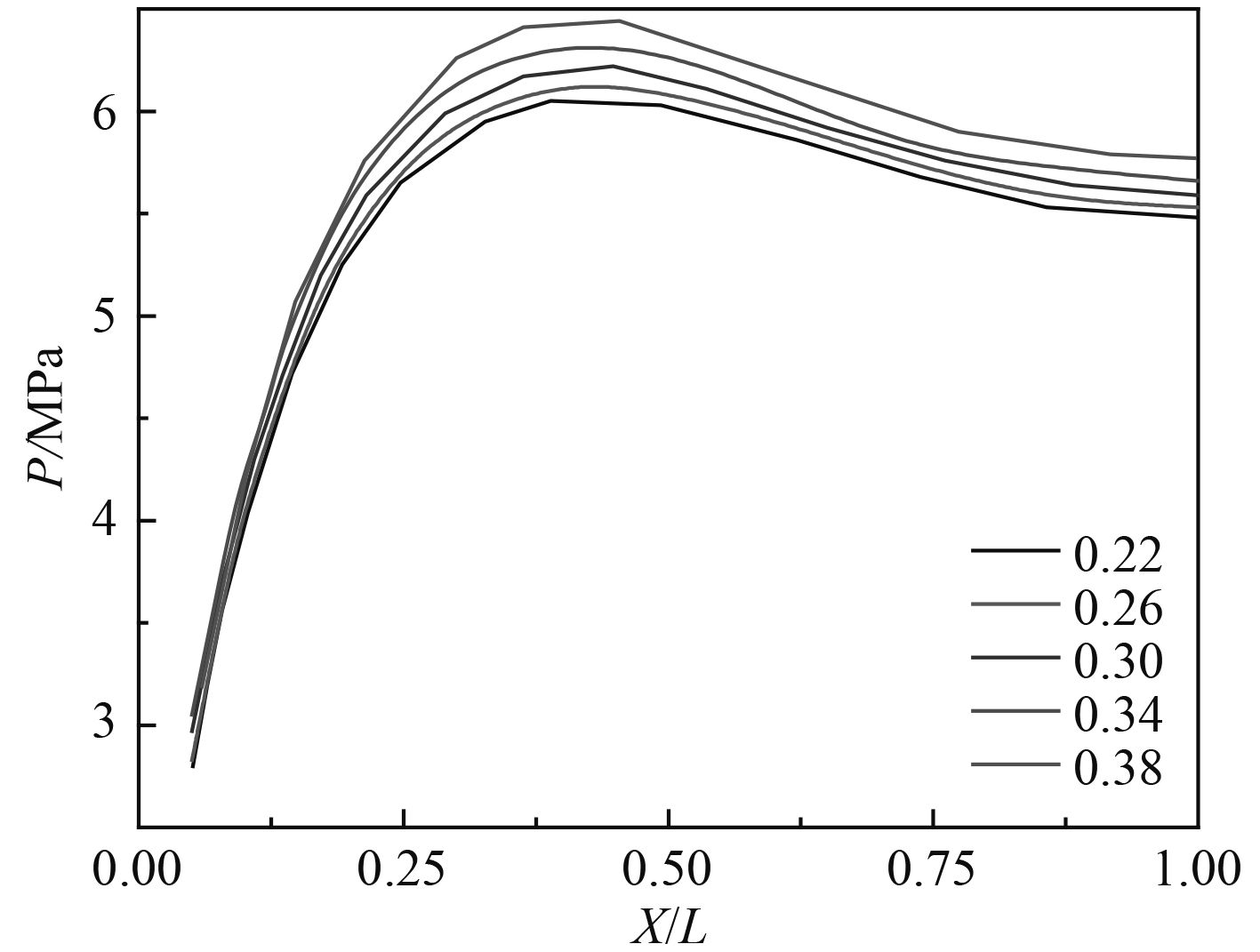图 13 不同泊松比对冲击压力的影响 Fig. 13 Impact of different Poisson ratios on pressure表 3 不同泊松比对冲击压力的影响 Tab.3 Impact of different poisson ratios on pressure
3.4 结构入水速度对冲击压力的影响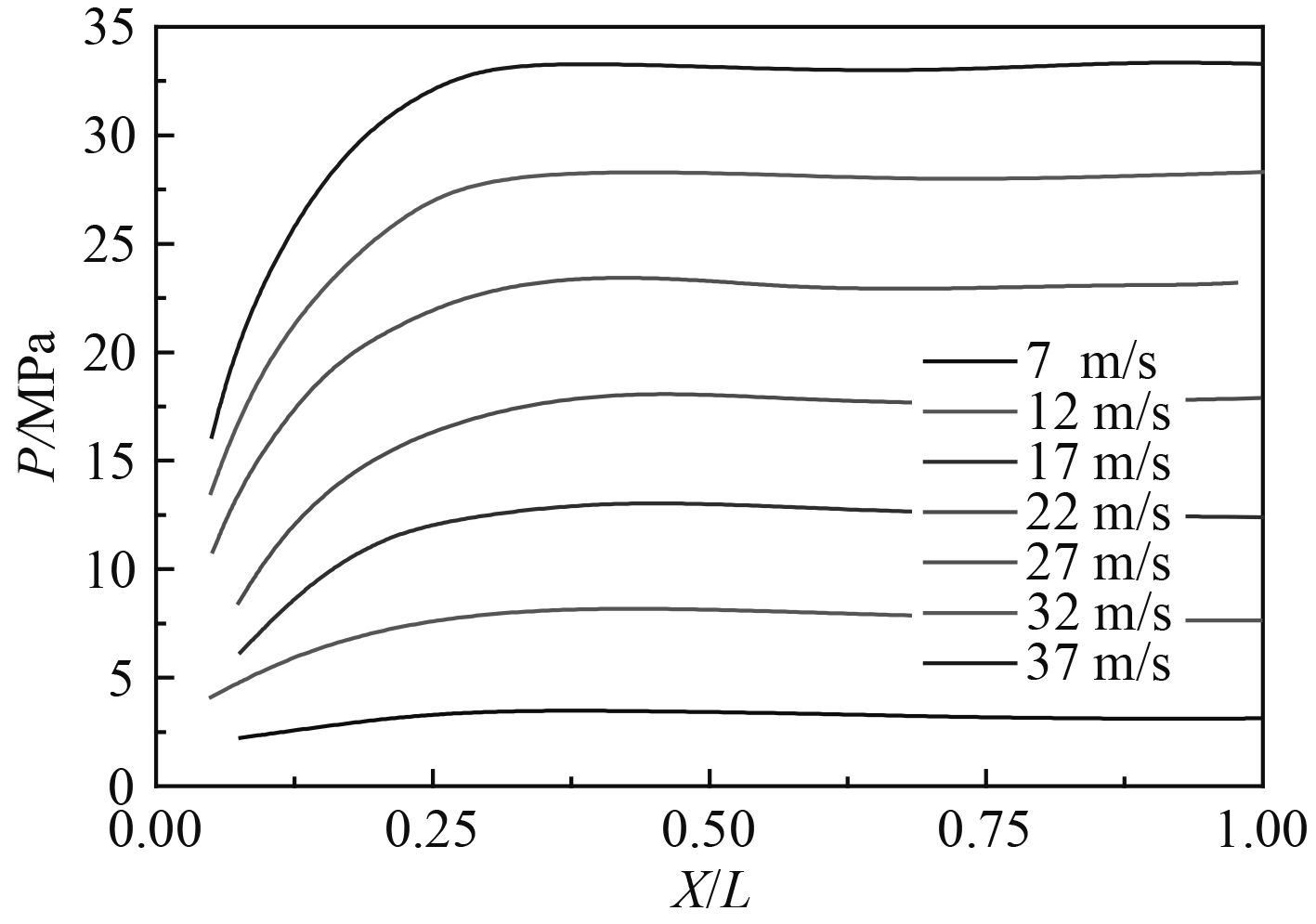图 14 入水速度对冲击压力的影响 Fig. 14 Influence of water inlet velocity on pressure表 4 入水速度对冲击压力的影响 Tab.4 The impact of water velocity on pressure
3.5 结构底部斜升角对冲击压力的影响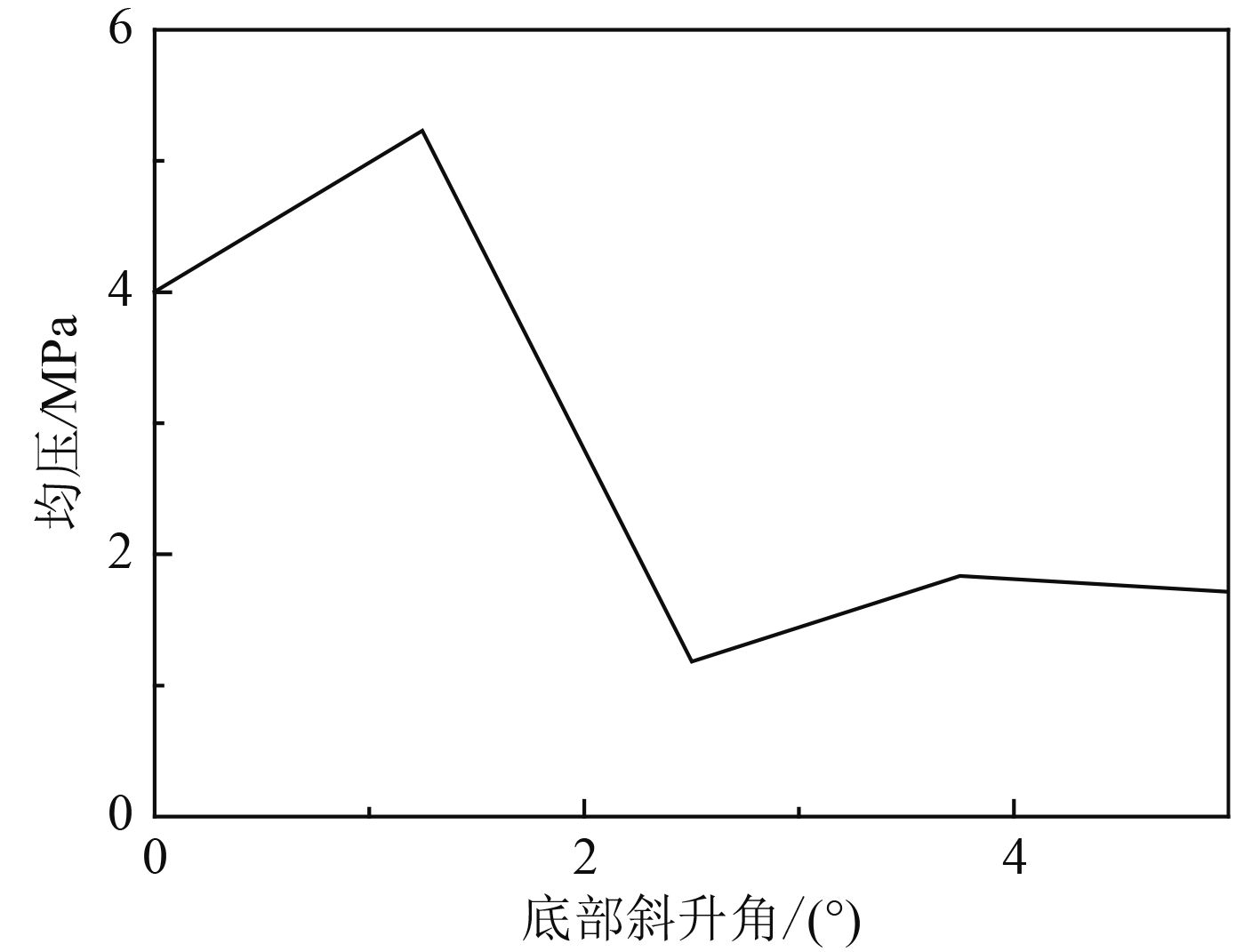图 15 不同底部斜升角入水冲击的均压值 Fig. 15 Pressure value of different bottom inclined angle into water
4 结　语

1）平底结构入水冲击过程中，底部边缘处最先达到峰值，随后沿宽度方向向中心依次出现压力峰值。底部中心区域（X/L=0～0.6）形成空气垫，空气垫对该区域的冲击产生缓冲作用。其缓冲作用在中心点处最明显，并沿宽度方向向两侧减弱，在X/L=0.6处为空气垫的边缘，出现压力最大值。

2）结构等速冲击入水后的运动为自由振动。冲击压力的峰值随结构质量的增大而增大。不同质量下底部均压的峰值几乎出现在同一时刻，压力分布曲线的形状基本一致，质量越小整个底部的压力值均越小。同时，质量越小空气垫的作用越明显，空气垫边缘的位置会随结构质量的增大向底部中心移动。

3）冲击压力的峰值随刚度的增大而增大。不同刚度下底部均压峰值出现的时刻随刚度的减小而顺延，冲击作用时间的长短会随弹性模量的减小而延长，压力分布曲线的形状基本保持一致。同时，刚度越大空气垫的缓冲作用越明显，且不同刚度下空气垫的边缘位置几乎不变。

4）冲击压力峰值与速度呈线性关系，随速度的增大而增大。速度对底部压力分布情况的影响并不显著。

5）底部斜升角对冲击压力峰值的影响十分显著。0°～2°时冲击压力峰值随底部斜升角的增大而增大，2°～3°时随底部斜升角的增大而减小，3°之后其值趋于稳定。

  KARMAN TV The impact of seaplane floats during landing[R]. Washington DC, USA: National Advisory Committee for Aero-nautics, NACA Technical Notes 321, 1929.  WAGNER V H. Phenomena associated with impacts and sliding on liquid surfaces[J]. Z Angew Math Mech, 1932, 12 (4): 193–215. DOI: 10.1002/(ISSN)1521-4001  WORTHINGTON A M. Impact with a liquid surface studied with aid of instantaneous photography[J]. Philosophical Transactions of the Royal Society of London, 1900, 194A : 175–199.  S. Experiments on flat-bottom slamming[J]. Journal of Ship Research, 1966, 10 (1): 10–27.  黄震球, 张文海. 减小平底体砰击的试验研究[J]. 武汉造船工程学会年会论文集(下), 1985 : 245–252.  李国钧, 黄震球. 平底物体对水面的斜向冲击[J]. 华中理工大学学报, 1995, 23 (1): 145–147.  卢炽华, 何友声. 二维弹性结构入水冲击过程中的流固耦合效应[J]. 力学学报, 2000, 36 (2): 129–140.  夏斌, 陈震, 肖熙. 弹性平底海洋结构物入水冲击的仿真分析[J]. 中国海洋平台, 2005, 20 (1): 22–28.  陈震, 肖熙. 平底结构砰击压力峰值分析[J]. 上海交通大学学报, 2006, 40 (6): 983–987.  陈震, 肖熙. 平底结构砰击压力的分布[J]. 中国造船, 2005, 46 (4): 97–103.  陈震, 肖熙. 空气垫在平底结构入水砰击中作用的仿真分析[J]. 上海交通大学学报, 2005, 39 (5): 670–673.  陈震, 肖熙. 基于神经网络的平底结构砰击压力预报[J]. 海洋工程, 2005, 23 (2): 26–31.  MATEJ V, STPHAN M, HEINER M, et al. Fluid models in LS-DYNA and their interaction with a structure in dynamic simulations[C]// ASME Pressure Vessels and Piping Division Conference. 2005.  OGER G, DORING M, ALESSANDRINI B, et al. Two-dimensional SPH simulations of wedge water entries[J]. Journal of Computational Physics, 2005, 213 : 803–822.  GONG Kai, LIU Hua, WANG Benlong. Water entry of a wedge based on SPH model with an improved treatment[J]. Journal of hydrodynamics, 2009, 21 (6): 750–757. DOI: 10.1016/S1001-6058(08)60209-7  吴家鸣, 龚国围, 朱良生. 平底结构砰击作用下限制水域的流体动力特性[J]. 华南理工大学学报, 2008, 36 (10): 97–107. DOI: 10.3321/j.issn:1000-565X.2008.10.020  HU Xiao-zhou, RAO Qiu-hua, ZHENG Hong-qiang. Numerical simulation of flatted-bottom seafloor mining tool and water entry process[C]// Fifth Conference on Measuring Technology and Mechatronics Automation. 2013.